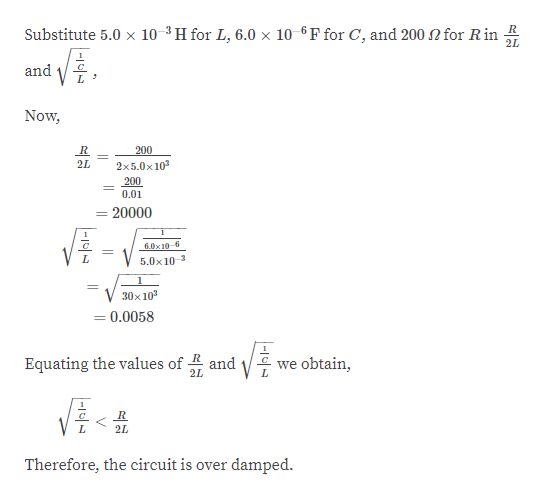# In an RLC circuit, L = 5.0 mH, C = 6.0μF, and R = 200 Ω.(a) Is the circuit underdamped, critically damped, or overdamped?(b) If the circuit starts oscillating with a charge of 3.0 × 10−3 C on the capacitor, how much energy has been dissipated in the resistor by the time the oscillations cease?

Question
34 views

In an RLC circuit, L = 5.0 mH, C = 6.0μF, and R = 200 Ω.

(a) Is the circuit underdamped, critically damped, or overdamped?

(b) If the circuit starts oscillating with a charge of 3.0 × 10−3 C on the capacitor, how much energy has been dissipated in the resistor by the time the oscillations cease?

check_circle

Step 1

Given information:

Resistance (R) = 200 ohm

Inductance (L) = 5.0 mH

Capacitance (C) = 6.0 µF

Step 2
Step 3help_outlineImage TranscriptioncloseSubstitute 5.0 x 10-³H for L, 6.0 × 10-6 F for C, and 200 N for Rin 2 and Now, 200 2L 2x5.0x10 200 0.01 20000 6.0x10 6 5.0x10 3 30x 10 = 0.0058 Equating the values of R and E we obtain, 2L 2L Therefore, the circuit is over damped. |-14|4 fullscreen

### Want to see the full answer?

See Solution

#### Want to see this answer and more?

Solutions are written by subject experts who are available 24/7. Questions are typically answered within 1 hour.*

See Solution
*Response times may vary by subject and question.
Tagged in

### Electromagnetic Induction